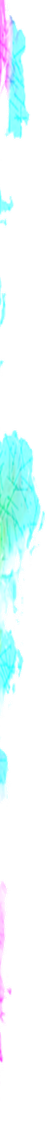### RegExp Group & References

• turned_in_notCharacter Sets
• (x)
• (?:x)
• x|y
• \N
• To run below examples, try it inside `<script>` tag, in a basic html file

##### (x)

It matches x & remembers the match, which are access by RegExp.\$N, where N is any positive number

• The number of '(x)' used in your regular expression is the maximum value of 'N' (but limited till 9) you can access
• Note! If more than 9 '(x)' used then we will access them with the help of `exec()` covered under RegExp methods

For example :

``````var str = "black book";
var pattern = /(b.)./g;
// Output: bla,boo
// Output: bo
``````

Matches: black book

Comment : RegExp.\$1 remembers the last match via (b.) which is 'bo' in above case

Syntax - To access the remember match
``RegExp.\$N   // 10 > N > 0``
Syntax - To access the remember match length
``RegExp.\$N.length``
Syntax - To access the remember match via index value
``RegExp.\$N[index]  // index starts from 0``

##### (?:x)

It matches x but don't remember the match

For example :

``````var str = "black book";
var pattern = /(?:b.)./g;
// Output: bla,boo
// Output:
``````

Matches: black book

Comment : It doesn't remember match via (?:b.)

##### x|y

It matches either x or y

• Use (x|y) format if you have to integrate with other characters, like: /a(b|c|d)/ looks for 'ab' or 'ac' or 'ad'

For example :

``````var str = "black book, red book";
var pattern = /black|red/g;
// Output: black,red``````

Matches: black book, red book

##### \N

It holds the reference of the match value by (x), where N is any positive number

For example :

``````var str = "pen! yes,paper! yes,book! no";
var pattern = /pen(! )(yes,)paper\1\2/g;